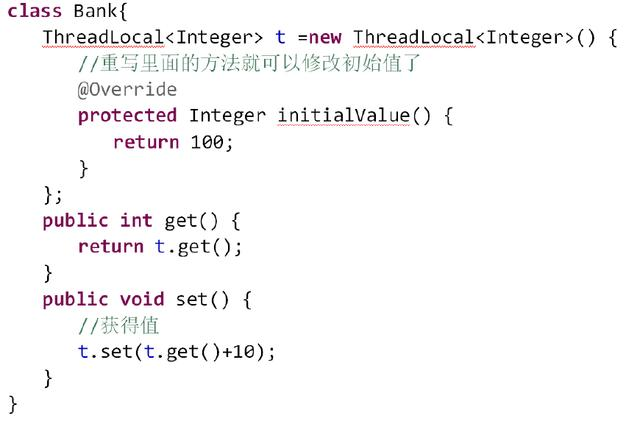ThreadLocal中的值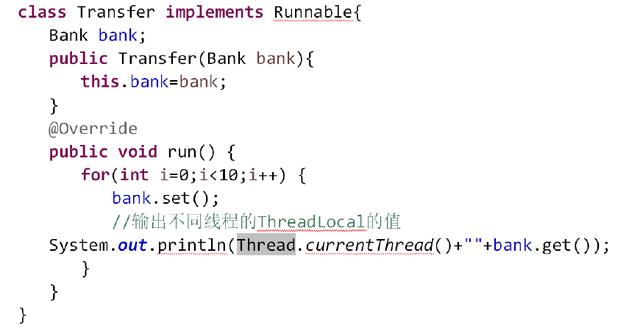对ThreadLocal的操作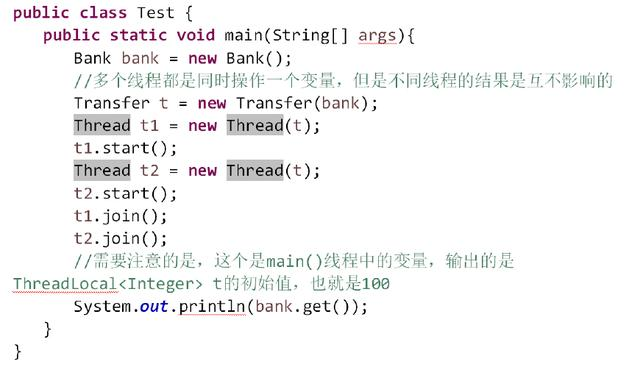创建线程并等待线程执行完成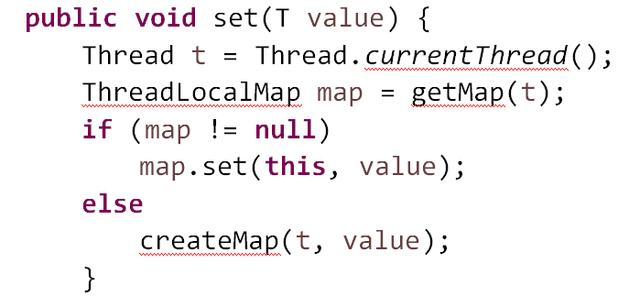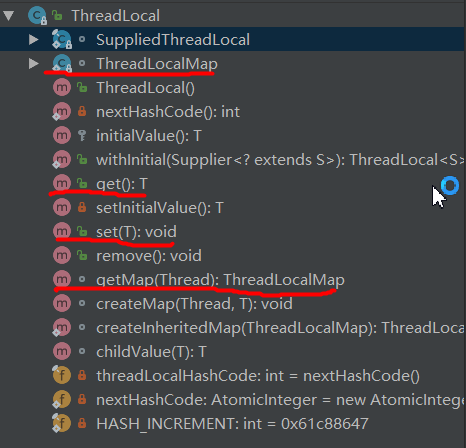``````    /* ThreadLocal values pertaining to this thread. This map is maintained by the ThreadLocal class. */
``````

``````        static class Entry extends WeakReference<ThreadLocal<?>> {
/** The value associated with this ThreadLocal. */
Object value;

super(k);
value = v;
}
}
``````

``````    public void set(T value) {
if (map != null)
map.set(this, value);
else
createMap(t, value);
}

public T get() {
if (map != null) {
if (e != null) {
@SuppressWarnings("unchecked")
T result = (T)e.value;
return result;
}
}
return setInitialValue();
}
``````

``````    ThreadLocalMap getMap(Thread t) {
}
``````

``````    void createMap(Thread t, T firstValue) {
}
``````

``````public class Son implements Cloneable{
public static void main(String[] args){
Son p=new Son();
System.out.println(p);
public void run(){
try {
} catch (CloneNotSupportedException e) {
e.printStackTrace();
}
}});
t.start();
}
}
``````

``````Son@7852e922
Son@7852e922
Son@313b781a
``````

``````private final int threadLocalHashCode = nextHashCode();
private static AtomicInteger nextHashCode = new AtomicInteger();
private static final int HASH_INCREMENT = 0x61c88647;
private static int nextHashCode() {
}
``````

``````public class Son implements Cloneable{
public static void main(String[] args){
public void run(){
}});
t.start();
}
}
``````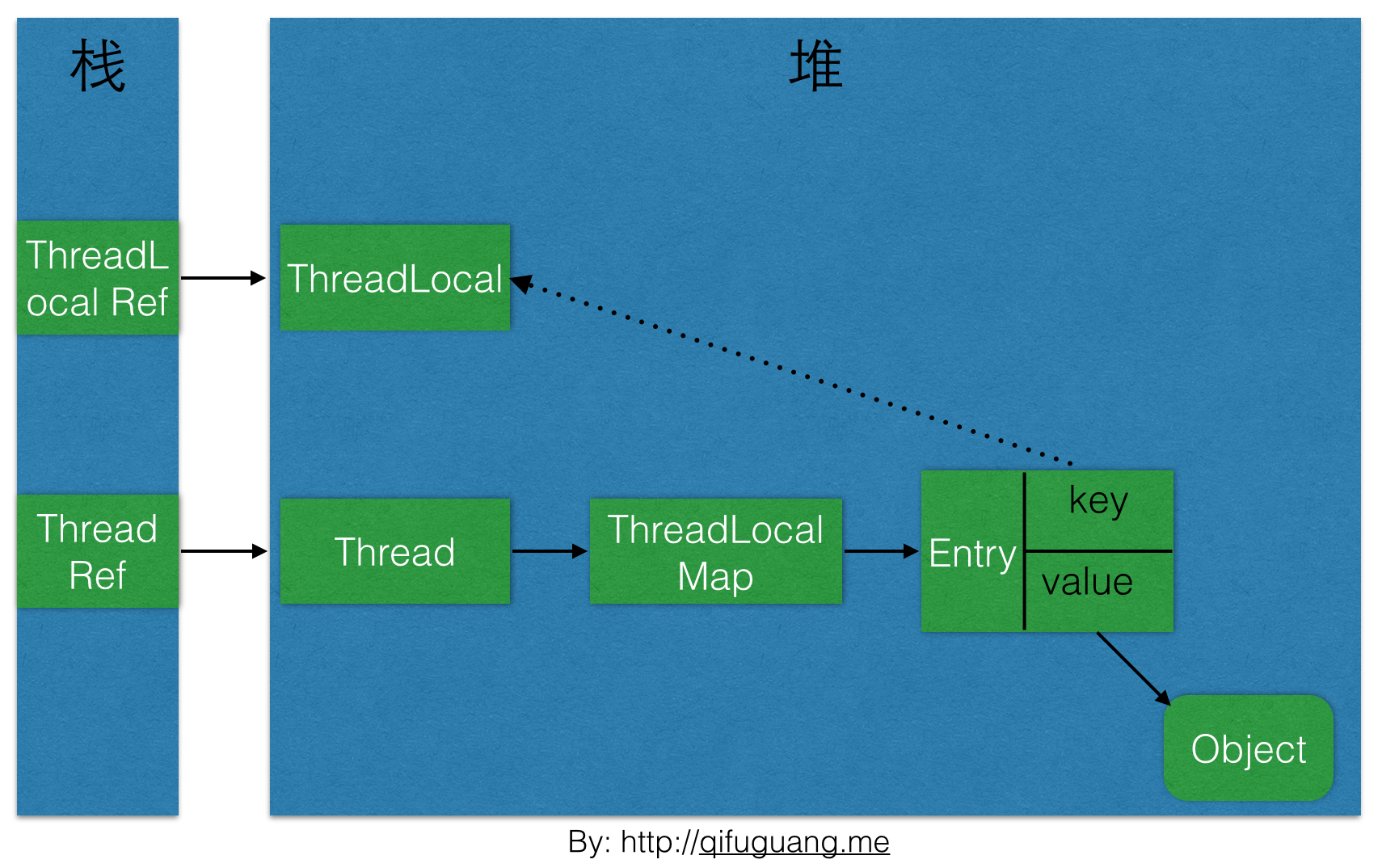2. 如果e为null或者key不一致则向下一个位置查询，如果下一个位置的key和当前需要查询的key相等，则返回对应的Entry。否则，如果key值为null，则擦除该位置的Entry，并继续向下一个位置查询。在这个过程中遇到的key为null的Entry都会被擦除，那么Entry内的value也就没有强引用链，自然会被回收。仔细研究代码可以发现，set操作也有类似的思想，将key为null的这些Entry都删除，防止内存泄露。

## 理解

``````class Data {
public Integer count = 0;

public Integer getNumber() {
return ++count;
}

}

private Data data;

this.data = data;
}

@Override
public void run() {
for (int i = 0; i < 3; i++) {
}
}

public static void main(String[] args) {
Data data= new Data();
t1.start();
t2.start();
}
}
``````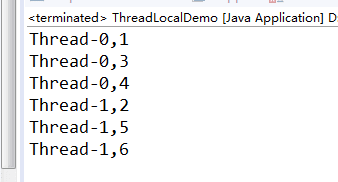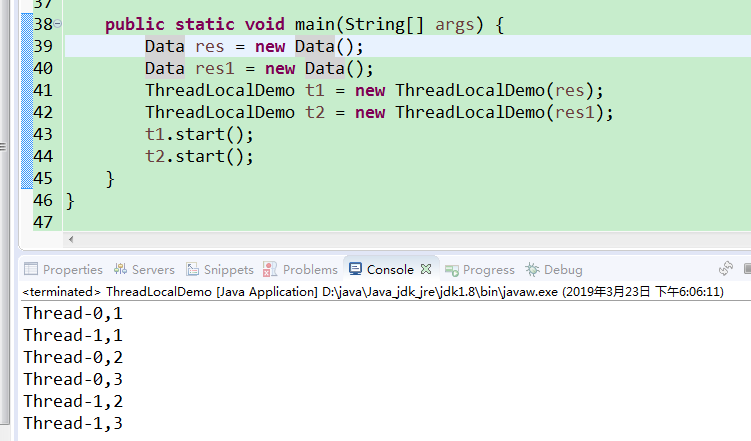void set(Object value)设置当前线程的线程局部变量的值。
public Object get()该方法返回当前线程所对应的线程局部变量。
public void remove()将当前线程局部变量的值删除，目的是为了减少内存的占用，该方法是JDK 5.0新增的方法。需要指出的是，当线程结束后，对应该线程的局部变量将自动被垃圾回收，所以显式调用该方法清除线程的局部变量并不是必须的操作，但它可以加快内存回收的速度。

``````class Data {

protected Integer initialValue() {
return 0;
};
};

public Integer getNumber() {
int count = threadLocal.get() + 1;
return count;

}

}

private Data data;

this.data = data;
}

@Override
public void run() {
for (int i = 0; i < 3; i++) {
}
}

public static void main(String[] args) {
Data res = new Data();
t1.start();
t2.start();
}
}
``````

`````` static class Entry extends WeakReference<ThreadLocal<?>> {

Object value;
super(k);
value = v;
}
}
``````

1、set()

``````    public void set(T value) {
if (map != null)
map.set(this, value);
else
createMap(t, value);
}
``````

``````      ThreadLocalMap(ThreadLocal<?> firstKey, Object firstValue) {
table = new Entry[INITIAL_CAPACITY];
int i = firstKey.threadLocalHashCode & (INITIAL_CAPACITY - 1);
table[i] = new Entry(firstKey, firstValue);
size = 1;
setThreshold(INITIAL_CAPACITY);
}
``````

`````` private void set(ThreadLocal<?> key, Object value) {
Entry[] tab = table;
int len = tab.length;
//定位hash桶的位置
int i = key.threadLocalHashCode & (len-1);
//发生hash碰撞时如果碰撞的位置上已经有Entry，且原有的key没有被回收，就查找数组下一个位
for (Entry e = tab[i];
e != null;
e = tab[i = nextIndex(i, len)]) {
//Key存在就替换原来的value值
if (k == key) {
e.value = value;
return;
}
//key为空就替换并清除过期的Entry
if (k == null) {
replaceStaleEntry(key, value, i);
return;
}
}
//在空的位置上放入Entry之前先判断是否需要扩容
tab[i] = new Entry(key, value);
int sz = ++size;
if (!cleanSomeSlots(i, sz) && sz >= threshold)
rehash();
}
``````

2、get（）

``````public T get() {
if (map != null) {
if (e != null) {
@SuppressWarnings("unchecked")
T result = (T)e.value;
return result;
}
}
return setInitialValue();
}
``````

``````/**
*/

private T setInitialValue() {
T value = initialValue();
if (map != null)
map.set(this, value);
else
createMap(t, value);
return value;
}
``````

`````` private Entry getEntry(ThreadLocal<?> key) {
int i = key.threadLocalHashCode & (table.length - 1);
Entry e = table[i];
if (e != null && e.get() == key)
return e;
else
return getEntryAfterMiss(key, i, e);
}
``````

``````private Entry getEntryAfterMiss(ThreadLocal<?> key, int i, Entry e) {
Entry[] tab = table;
int len = tab.length;
//从当前位置向下寻找
while (e != null) {
//相同就直接返回结果
if (k == key)
return e;
//如果为null调用expungeStaleEntry()处理
if (k == null)
expungeStaleEntry(i);
//继续寻找下一个位置
else
i = nextIndex(i, len);
e = tab[i];
}
//最后没找到返回NULL
return null;
}
``````

3、remove（）

``````  private void remove(ThreadLocal<?> key) {
Entry[] tab = table;
int len = tab.length;
int i = key.threadLocalHashCode & (len-1);
for (Entry e = tab[i];
e != null;
e = tab[i = nextIndex(i, len)]) {
if (e.get() == key) {
e.clear();
expungeStaleEntry(i);
return;
}
}
}
``````

### 使用方式一

``````public class TestThreadLocal {

//线程本地存储变量
@Override
protected Integer initialValue() {
return 0;
}
};

public static void main(String[] args) {
for (int i = 0; i < 3; i++) {//启动三个线程
@Override
public void run() {
}
};
t.start();
}
}

/**
* 线程本地存储变量加 5
*/
for (int i = 0; i < 5; i++) {
n += 1;
}
}

}
``````

``````Thread-1 : ThreadLocal num=1
``````

### 使用方式二

``````import java.util.concurrent.ExecutorService;
import java.util.concurrent.Executors;

//线程本地存储变量
@Override
protected Integer initialValue() {
return 0;
}
};

public static void main(String[] args) {
for (int i = 0; i < 10; i++) {
}
}

/**
* 线程本地存储变量加 5
*/
for (int i = 0; i < 5; i++) {
n += 1;
}
}

}
``````

``````pool-1-thread-1 : ThreadLocal num=1
``````

``````public T get() { }
public void set(T value) { }
public void remove() { }
protected T initialValue() { }
``````
• set()用来设置当前线程中变量的副本
• remove()用来移除当前线程中变量的副本
• initialValue()是一个protected方法，一般是用来在使用时进行重写的，如果在没有set的时候就调用get，会调用initialValue方法初始化内容。

## 源码分析

###### set
``````     public void set(T value) {
if (map != null)
map.set(this, value);
else
createMap(t, value);
}
``````

###### getMap
``````    ThreadLocalMap getMap(Thread t) {
}
``````

###### createMap
``````void createMap(Thread t, T firstValue) {
}
``````

###### get
``````    public T get() {
if (map != null) {
if (e != null) {
@SuppressWarnings("unchecked")
T result = (T)e.value;
return result;
}
}
return setInitialValue();
}
``````

###### remove
``````    public void remove() {
if (m != null)
m.remove(this);
}
``````

## 使用场景

2. 比如用来存储用户 Session。Session 的特性很适合 ThreadLocal ，因为 Session 之前当前会话周期内有效，会话结束便销毁。